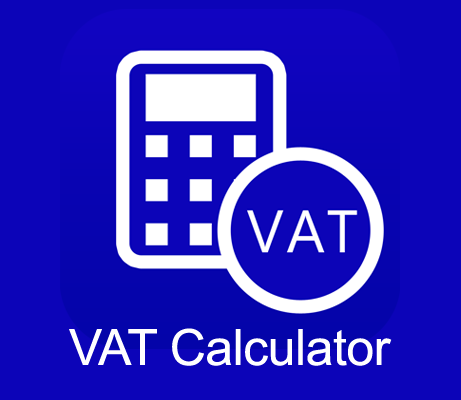# VAT Calculator

Whether you are adding VAT to a price, subtracting VAT from a given price or calculating the VAT amount, this VAT calculator can save you time.
- This handy tool helps you calculate the price with VAT (or gross price), price without VAT (or net price) and the VAT amount.
- Unlike many other VAT calculators, this one supports fractional values (input fractions in the following format: 2/3, 3_1/2, etc).
- In addition, you can view recent VAT calculations and share results via email.

## How to calculate VAT

Value Added Tax (VAT) is charged on most of the goods and services we buy. Whether you have to add VAT to get the final price of products or services or you need to subtract VAT to know the price without VAT, VAT calculator helps you with all VAT calculations. Just enter the VAT rate and either the gross or the net price and the VAT calculator will work out the rest.

VAT Calculator details:
Current version: VAT Calculator 1.0.0

### How to add or subtract VAT using the VAT Calculator

#### Adding VAT to a price

- select "Calculate price with VAT"
- enter the net price and the VAT rate (varies between contries)
- click "Calculate"
This will let you know the price with VAT and the amount of VAT.

For example, the price without VAT is 180 euros and the VAT rate is 21%. You need to calculate the amount of VAT and the total price with VAT.
- select "Calculate price with VAT"
- enter price without VAT = 180 EUR
- enter VAT rate = 21%
- click "Calculate"
The calculator shows: price with VAT = 217.8 EUR, VAT amount = 37.8 EUR

#### Subtracting VAT from the gross price

- select "Calculate price without VAT"
- input the total price and the VAT rate
- click "Calculate"
The calculator will show you the price without VAT and the amount of VAT.

For example, the gross price is 320 euros, the VAT rate is 15% and you want to find out the price before VAT.
- select "Calculate price without VAT"
- enter price with VAT = 320 EUR
- enter VAT rate = 15%
- click "Calculate"
The calculator will show the net price and the amount of VAT:
price without VAT = 278.26 EUR
VAT amount = 41.74 EUR

#### How do I calculate VAT? What formula do I use to work out the VAT?

If you know a net price and VAT rate but want to find out the price after tax:
If we assume that VAT rate is 20%, then gross price = net price x 1.2

If you know a gross price but want to find out the price before tax:
If we assume that VAT rate is 18%, then net price = gross price ÷ 1.18

#### How to calculate VAT amount

Example 1. The price before VAT is 130 euros, the VAT rate is 19%. How to work out the VAT and the price including VAT.
total price = net price x 1.19 => total price = 130 x 1.19 = 154.7
VAT amount = total price - net ptice => VAT amount = 154.7 - 130 = 24.7

Example 2. Sometimes you have a total price and need to find the amount of VAT it contains. For example, the gross price is 120 euros, the VAT rate is 21% and you want to calculate the amount of VAT.
net price = total price ÷ 1.21 => net price = 120 ÷ 1.21 = 99.17
VAT amount = total price - net price => VAT amount = 120 - 99.17 = 20.83